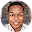# BECE 2014 Mathematics Paper 1OBJECTIVE TEST

Question 1: If set N is a subset of set M, then
A. sets M and N have the same number of elements.
B. some members of set N can be found in set M.
C. no member of set N is in set M.
D. all members of set N are in set M.

Question 2:

Question 3:
Question 4: Simplify: 12 - 7 - (-5)
A. -10
B. -2
C. 0
D. 10

Question 5: Express 72 as a product of its prime factors.
A. 2 x 3³
B. 2² x 3³
C. 2³ x 3
D. 2³ x 3²

Question 6: Find the smallest number which is divisible by 16 and 20?
A. 40
B. 80
C. 120
D. 160

Question 7: Convert 243[base five] to a base ten numeral.
A. 40
B. 43
C. 45
D. 73

Question 8: A pineapple which was bought for GH¢1.00 was sold at GH¢1.30. Calculate the profit percent
A. 10%
B. 20%
C. 23%
D. 30%

Question 9: Simplify 35x⁵y³ ÷ 7xy²
A. 5x⁴y
B. 5x⁴y⁵
C. 5x⁶y
D. 5x⁶y⁵

Question 10: Two bells P and Q ring at intervals of 3 hours and 4 hours, respectively. After how many hours will the two bells first ring simultaneously (at the same time)?
A. 6 hours
B. 8 hours
C. 12 hours
D. 24 hours

Question 11: A boy scores ¹⁷∕₂₅ in a French test. Express his score as a percentage.
A. 17%
B. 34%
C. 68%
D. 85%

Question 12: Arrange the following fractions in ascending order of magnitude,²∕₅, ⁵∕₁₂ and ¾.
A. ²∕₅, ¾, ⁵∕₁₂
B. ²∕₅, ⁵∕₁₂, ¾
C. ⁵∕₁₂, ²∕₅, ¾
D. ¾, ²∕₅, ⁵∕₁₂

Question 13: Kofi paid rent of GH¢1,800.00 each year. If the rent is 0.3 of his annual income, find his annual income.
A. GH¢600.00
B. GH¢5,400.00
C. GH¢6,000.00
D. GH¢18,000.00

Question 14: I gave a storekeeper a GH¢10.00 note for goods I bought. He asked me for another 15 Gp for ease of change. If he then gave me 50Gp, how much did I pay for the goods?
A. GH¢9.35
B. GH¢9.45
C. GH¢9.65
D. GH¢10.65

Question 15: Kojo can buy 15 shirts at GH¢4.00 each. If the price is increased to GH¢5.00, how many shirts can he now buy?
A. 12
B. 15
C. 19
D. 20

Question 16: A hall which is 8 m long is represented on a diagram as 4 cm long. What is the scale of the diagram?
A. 1 : 200
B. 1 : 250
C. 1 : 400
D. 1 : 800

Question 17: Jane arrived at work at 7:55 a.m. and left at 4:15 p.m. For how long was she at work?
A. 7 hr 20 min
B. 7 hr 45 min
C. 8 hr 20 min
D. 8 hr 40 min

Question 18: Given that (3.14 x 18) x 17.5 = 3.14 x (3p x 17.5)
Find the value of p.
A. 3.0
B. 5.8
C. 6.0
D. 9.0

Question 19: Question 20: Question 21: Question 22: In an enlargement with scale factor 2, which of the following statements is not true?
A. Each length is multiplied by 2.
B. Each angle remains the same.
C. The shape of the figure does not change.
D. The size of the figure does not change.

Question 23: Kofi, Kojo and Ama shared GH¢480,000.00 in the ratio 3 : 5 : 4. How much did Ama receive?
A. GH¢160,000.00
B. GH¢200,000.00
C. GH¢218,181.81
D. GH¢342,859.14

Question 24: If w = 12, x = 5, y = 6 and z = 4, find the value of wx – yz.
A. 18
B. 27
C. 36
D. 84

Question 25: A man was 24 years old when his son was born. Now he is three times as old as his son. Find the age of the son.
A. 6 years
B. 12 years
C. 18 years
D. 36 years

Question 26: There are 20 identical balls in a box. Twelve are blue and the rest are green. If one ball is taken at random from the box, find the probability that the ball is green.
A. ⅟₂₀
B. ⅖
C. ⅗
D. ¾

Question 27: Question 28: The perimeter of rectangle is 24cm. If the length is 7cm, find its width.
A. 3 cm
B. 5 cm
C. 10cm
D. 12cm

Question 29: Question 30: How many faces has a cube?
A. 4
B. 6
C. 8
D. 12

Question 31: The diameter of a circular tray is 28 cm. Find the area of the tray. [Take π = ²²∕₇]
A. 44cm²
B. 88cm²
C. 154cm²
D. 616cm²

Question 32: Calculate the volume of a cylinder with radius 7 cm and height 10 cm.[Take π = ²²∕₇]
A. 220cm²
B. 440cm²
C. 1,540cm²
D. 3,080cm²

Question 33: Question 34: Question 35: A 3.6 m long string is to be cut into pieces, each of length 40 cm. How many pieces can be cut from the string?
A. 4
B. 6
C. 8
D. 9

Question 36: Question 37: The point P(5, 4) is reflected in the y-axis. Find its image.
A. (-5, 4)
B. (5, -4)
C. (-4, 5)
D. (4, -5)

Question 38: Question 39: Find the gradient of the line which passes through the points M( -1, 2) and N(6, -3).
A. -⁵∕₇
B. -⁷∕₅
C. ⁵∕₇
D. ⁷∕₅

Question 40: Find the next two terms in the sequence 11, 7, 3, -1, ......., .......
A. 5, 9
B. 3, 7
C. -4,-9
D. -5,-9